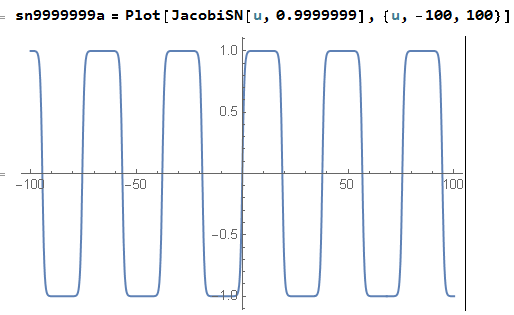### Jacobi Elliptic sn – the case of a stuttered sinus

In my last post I introduced Jacobi elliptic sinus, the functionwithreal, andOne has to be careful with notation here. Let us have a look at the definition as it is given on Wolfram’s pages:It is almost the same, but not the same as one from “Handbook of Mathematical Functions“, Ed. Milton Abramowitz and Irene A. Stegun:Here Abramowitz and Stegun write simply, but later on they use the notationwhereof Wolfram. Wolfram’s Mathematica is using JacobiSN(u,m). Maple is usingMatlab warns the user:We will useThe parameteris sometimes called the “modulus”. The shape of the function depends on the value ofWhenwe have just ordinary sinus:At the other extreme, forsn is nothing else but the hyperbolic tangent:In between, for, it is what I would dare to call a “stuttering sinus“. This stuttering is not seen at all forIt looks just as if the period became a little longer:and it is hard to notice forBut forwe getIt almost looks like the hyperbolic tangent, but when we zoom out we can see the stuttering:Clear case of stutteringThe graphs looks somewhat like that of a rectangular signal. There are “flips” and then there are longer and longer periods when the function is almost constant – between the flips. This is the main characteristic of Dzhanibekov effect: there are almost pure rotation periods, and sudden flips when the axis suddenly reverses the direction. But for all values ofthe function is periodic (forwe have an exception – we have infinite period).Forthe period isthen slowly grows, but forvery close toit becomes very sensitive to the value ofFor this reason every repetition of the Dzhanibekov effect as seen in the movies taken in space would probably give a different period.